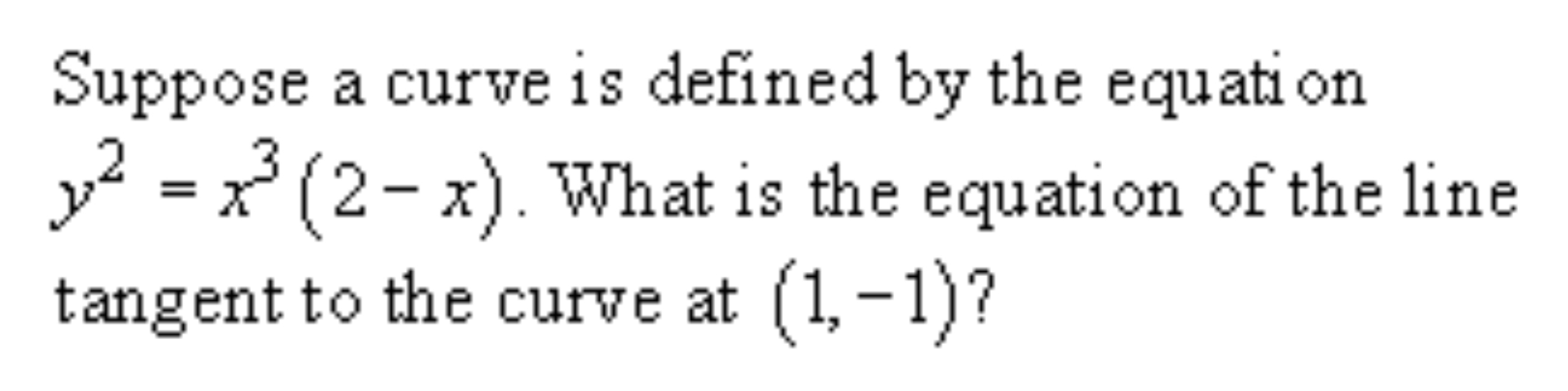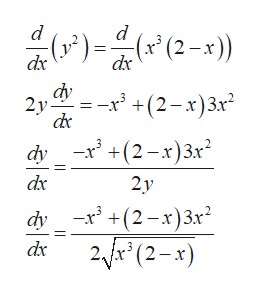# Suppose a curve is defined by the equati ony2 = x*(2- x). What is the equation of the linetangent to the curve at (1,-1)?

Question
6 viewshelp_outlineImage TranscriptioncloseSuppose a curve is defined by the equati on y2 = x*(2- x). What is the equation of the line tangent to the curve at (1,-1)? fullscreen
check_circle

Step 1

Given curve

Step 2

Given point on the curve (1, -1)

Step 3

To determine tangent to the given point of the curve

We determine tangent of the cur...help_outlineImage Transcriptionclose() (2-x)) dx dx r +(2-x)3x -x' +(2-x)3x 2y dy dx 2y dy x(2x)3x2 fA (2-x) dx fullscreen

### Want to see the full answer?

See Solution

#### Want to see this answer and more?

Solutions are written by subject experts who are available 24/7. Questions are typically answered within 1 hour.*

See Solution
*Response times may vary by subject and question.
Tagged in

### Functions